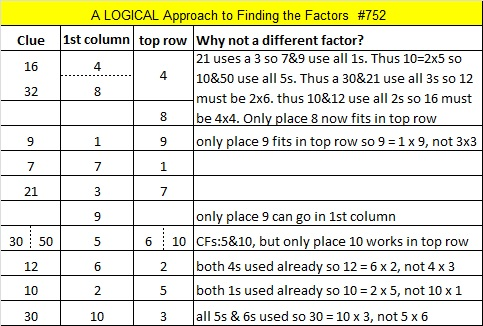# 752 and Level 6

• 752 is a composite number.
• Prime factorization: 752 = 2 x 2 x 2 x 2 x 47, which can be written 752 = (2^4) x 47
• The exponents in the prime factorization are 4 and 1. Adding one to each and multiplying we get (4 + 1)(1 + 1) = 5 x 2 = 10. Therefore 752 has exactly 10 factors.
• Factors of 752: 1, 2, 4, 8, 16, 47, 94, 188, 376, 752
• Factor pairs: 752 = 1 x 752, 2 x 376, 4 x 188, 8 x 94, or 16 x 47
• Taking the factor pair with the largest square number factor, we get √752 = (√16)(√47) = 4√47 ≈ 27.422618.Here’s today’s puzzle. A logical way to solve it is given in the table at the end of the post.Print the puzzles or type the solution on this excel file: 10 Factors 2016-01-18

—————————————

Here’s a few more thoughts about the number 752:

52 is divisible by 4 so 752 is also divisible by 4. However, 52 is not also divisible by 8, but since 7 is odd, 752 IS divisible by 8.

8 + 9 + 10 + 11 + 12 + 13 + 14 + 15 + 16 + 17 + 18 + 19 + 20 + 21 + 22 + 23 + 24 + 25 + 26 + 27 + 28 + 29 + 30 + 31 + 32 + 33 + 34 + 35 + 36 + 37 + 38 + 39 = 752; that’s 32 consecutive numbers.

752 is the sum of two consecutive primes: 373 + 379 = 752.

—————————————